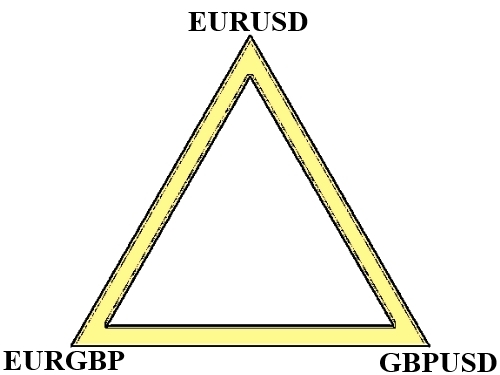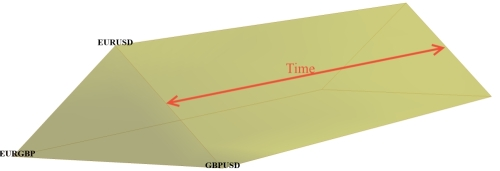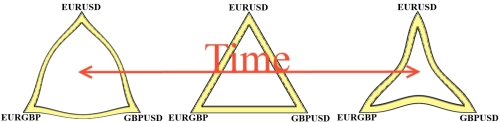In the market map, Forex is an archipelago.

PROXIMITY CONSTANT

At a quick glance we can see that the islands are related by proximity. This is evident, for example, for the pairs that share a base currency: EURUSD, EURGBP... As a result, movement in one of the islands affect the movement of the adjacent: the move of EURSD affect to EURGBP, EURJPY...

This relationship is mathematically explicit by a simple equation:

EURUSD = x * EURGBP

Where x is something that explains the relationship between EURUSD and EURGBP; in this case x = GBPUSD

That is, the price of a pair can be deduced through the product of the proximate pairs. This algebraic equality can be expressed geometrically:This equality is a constant that is deducted from the first axiom of the market: the no-arbitrage. Or, likewise: it’s impossible to obtain profitability without risk.

The above equality is what is known as a triangular position, but we can create all sorts of positions; for example, a square position:

EURJPY = EURGBP * GBPUSD * USDJPY

These algebraic equalities are much easier to see if we replace the name of the currency with a number:

EUR = 1
USD = 2
GBP = 3
JPY = 4

As a result of this replacement, the above equalities may be expressed as follows:

1/2 = 1/3 * 3/2
1/4 = 1/3 * 3/2 * 2/4

Now, as the set of rational numbers is a mathematical field such that the multiplication satisfies the commutative property and there is a neutral number to the product of fractions, then we can generate an infinite number of equalities that limit to zero:

1/5 = 1/3 * 3/2 * 2/4 * 4/5
1/6 = 1/3 * 3/2 * 2/4 * 4/5 * 5/6

Or, equally, we can generate an infinite number of geometric shapes whose area is getting closer to a circle.

Ultimately, this fact shows that all currencies saved each other a linear relationship (the correlation can be either positive or negative). I.e., there is a constant value. If in the previous article we talked about forex as a zero-sum game between traders: the sum of the profits and losses of all agents is a constant value equal to 0; in this article we will interpret forex as a zero-sum game among the currencies themselves: the sum of depreciation and appreciation of all currencies is a constant value which limits to 0.

PIP VARIABLE

The proximity constant can be economically exploited by means of on pip variation. The value of a pip is not a constant, neither pairs, nor between the same pair over time.

The pip value is expressed grosso modo as a function of the type 1/x. For example, for a standard lot it’s so:

If EURUSD = 1.39, then their pip = ((0.0001/1.39) * 100,000) = 7.2
If EURGBP = 0.83, then their pip = ((0.0001/0.83) * 100,000) = 12

The pip value isn’t linearly related to the quotation of the exchange rate: the higher price, the lower value of the pip; the higher value of the pip, lowest the quote. This behaviour is unique to forex, it can be visualized assuming that both, pip and price, keep an asymptotic behaviour; ergo: they tend to zero as its price increases:UNCERTAINTY SHARED

Let's go back to look at the map: what matters are not the islands, but tectonics. The movement of the islands is the visible result of deeper movements. Since we can’t see the movement of tectonic plates, we talk about them as a source of uncertainty. However, we assume that there is a deeper relationship between islands according to the tectonic plate that they settle. If the islands were related before through their proximity (correlation), we will now relate them in order to share or not any source of uncertainty (cointegration).

Taking the triangular relationship mentioned above and projected the time, then we have the following figure:However, this projection is not realistic: the intensity (or speed) of movement is smaller as the distance from the epicentre increases. Or, in other words: the map of the markets can’t be 100% explained by Euclidean geometry. What we have over time is a triangle shape changing by movements of contraction and expansion:In the previous article we talked about how we could take advantage of the divergence between objective value and subjective value. In this article the divergence is between: 1st) pip value and 2nd) the speed (or intensity) of the movement of the pair. As mentioned above, in the market it is impossible the arbitrage between asset prices; however, it is feasible to arbitrate volatility of the relations between assets. For example, in the case of the triangle the arbitration is between EURUSD asset and EURGBP-GBPUSD spread. In the square position, arbitrage is between EURJPY-USDJPY spread and GBPUSD-EURGBP spread.

We talked about arbitration because, in theory, our position has no risk. It’s what is known as neutral market because each currency has been bought and sold thereby cancelling each inherent risk:

Sell EURJPY = sell EUR and buy JPY

But there is a mismatch with pip value (prices taken at the time of writing):

EURJPY = 7
EURGBP = 12
GBPUSD = 6
USDJPY = 10

And, in turn, there is an inequality in volatility (values taken with a standard ATR at time of writing):

EURJPY = 0.0741
EURGBP = 0.0005
GBPUSD = 0.0005
USDJPY = 0.0564

As a result of such inequalities, the positions should launch into the market with a hedge ratio that satisfies the condition of neutral market.

CONCLUSION

After an earthquake always occurs the calm. Bet on it is a low risk profit. Or what is the same: the volatility can be interpreted by a sinusoidal model without information loss. Therefore, betting on regression to the mean is a low risk profit. However, it must be established for positions to ensure a neutral market for first, because we are not interested in the movement inherent to each island (an impossible to estimate variable), but the archipelago (a constant due to the nature of the zero-sum).
Translate to English Show original